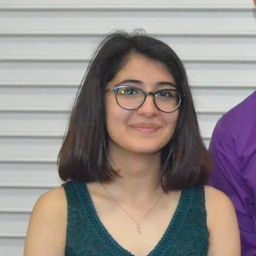# Bahar T.

Cornell University

## Biography

Hi! My name is Bahar Tehranipoor and I'm a student studying Computer Science at Cornell University. My favorite topics include calculus, statistics, and, of course, computer science!

## Education

BS Computer Science
Cornell University

## Topics Covered

Functions
Continuous Functions
Derivatives
Differentiation
Limits
Integrals
Integration
Integration Techniques
Applications of Integration
Differential Equations
Second-Order Differential Equations
Applications of the Derivative
Complex Numbers
Polynomials
Rational Functions
Equations and Inequalities
The Language of Algebra
Exponents and Polynomials
Exponential and Logarithmic Functions
Functions
Partial Derivatives
Functions of Several Variables
Fractions and Mixed Numbers
Decimals
The Integers
Series
Introduction to Sequences and Series
Introduction to Combinatorics and Probability
Parametric Equations
Polar Coordinates
Introduction to Conic Sections
Parallel and Perpendicular lines
Graphs and Statistics
Systems of Equations and Inequalities
Introduction to Matrices